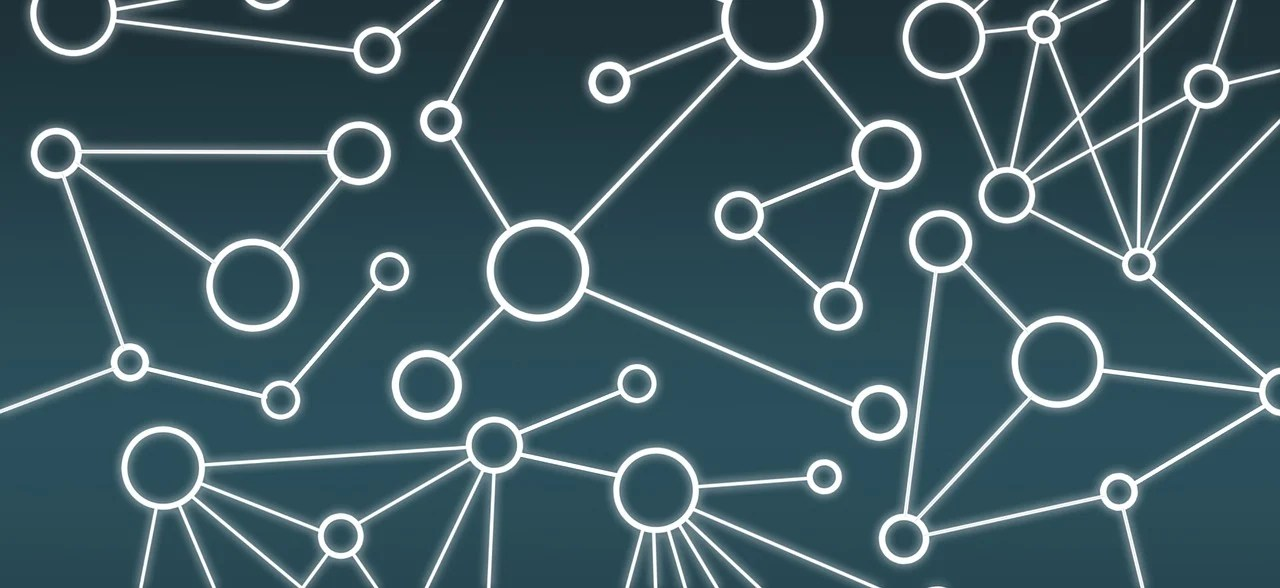# An introduction to graph states

#### What is a graph state?

One especially useful class of quantum states is graph states, also known as cluster states. As the name suggests, a graph state |G\rangle is associated with a graph,

G=(V,E),

where the vertices (v\in V) represent qubits initialised into the

|+\rangle = (|0\rangle+|1\rangle)/\sqrt{2}

state, and edges (e\in E) represent the application of controlled-phase (CZ) gates between respective vertices, where,

\mathrm{CZ}=\mathrm{diag}(1,1,1,-1).

A graph state can therefore be expressed as,

|G\rangle = \left[ \prod_{e\in E} \mathrm{CZ}_e \right] |+\rangle^{\otimes |V|}.

Since CZ gates are diagonal and therefore commute with one another, the order in which they are applied is irrelevant, meaning there is great flexibility in the preparation of graph states and room for parallelisation in the application of the required CZ gates.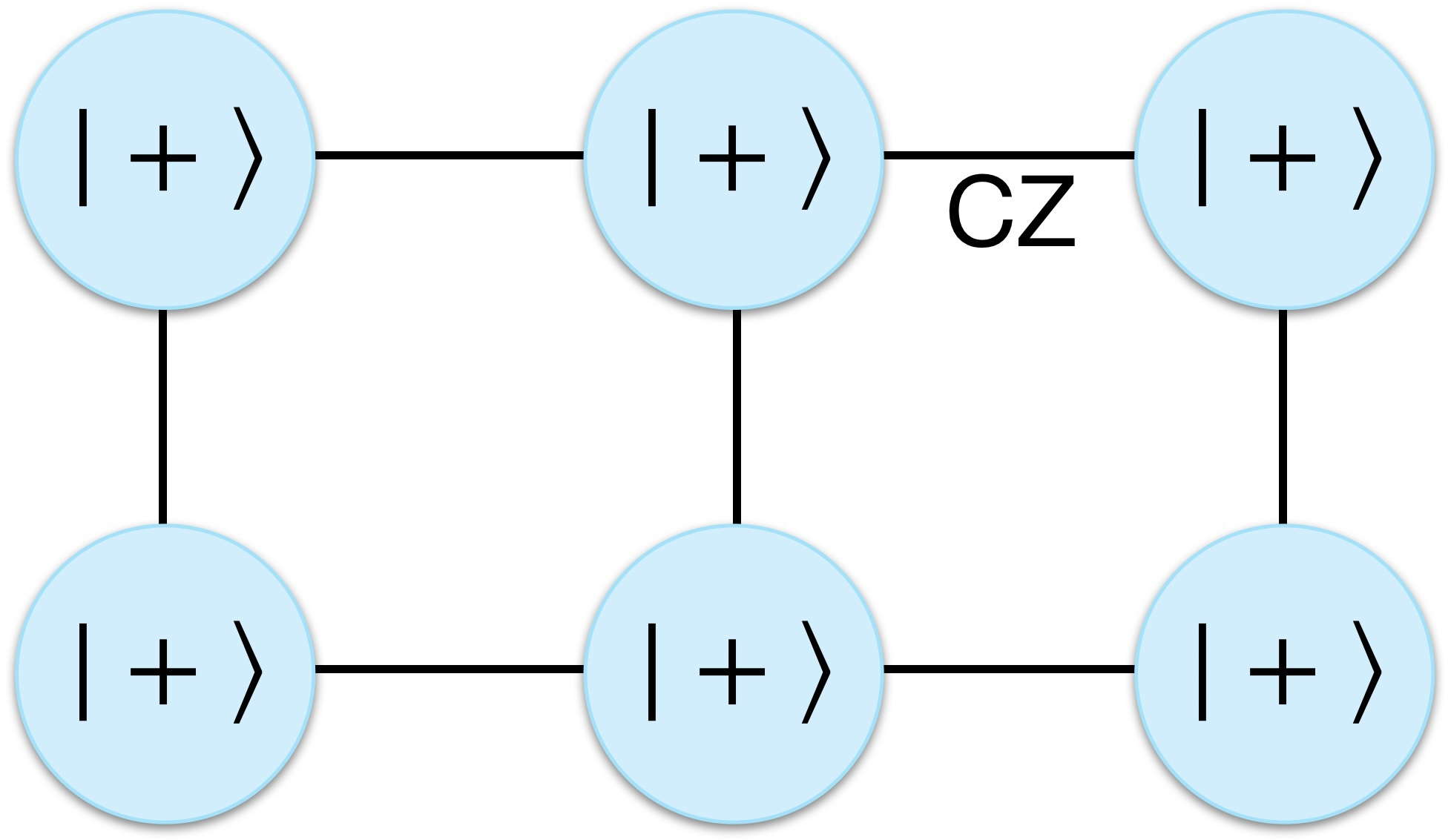A graph state is defined with respect to a graph, where vertices represent qubits initialised into the |+\rangle state and edges represent the application of CZ gates between qubits. Since CZ gates commute, the order in which they are applied is irrelevant.

An important special case is the two-qubit graph state, which is locally equivalent to a maximally-entangled Bell pair. From the definition, a two-qubit graph state can be written,

\mathrm{CZ}|+\rangle|+\rangle = \frac{1}{2}(|0,0\rangle + |0,1\rangle + |1,0\rangle - |1,1\rangle).

Applying a Hadamard gate to either qubit we obtain,

H\cdot \mathrm{CZ}|+\rangle|+\rangle = \frac{1}{\sqrt{2}}(|0,0\rangle + |1,1\rangle),

the |\Phi^+\rangle Bell pair.

Note that while a two-qubit graph state is maximally entangled, graph states, in general, are not, whose entanglement structure is defined over graph neighbourhoods, which in general are not global. The exceptions are star and fully connected graphs, both locally equivalent to GHZ states, where the entire graph forms a single neighbourhood.

#### Measurement-based quantum computing

Graph states are incredibly useful as they enable measurement-based quantum computing (MBQC) [Raussendorf, Browne & Briegel (2003)], an alternative way of thinking about quantum computation to the regular circuit model. In MBQC, computation proceeds only by measuring qubits from a graph state of some topology (usually a lattice), where the order and basis in which qubits are measured dictates the implemented computation. This model for computation has no classical analogue and is uniquely quantum.

The universality of MBQC using graph states is easiest to demonstrate by considering its equivalence with the circuit model. Following the narrative of Nielsen (2005), this can be seen by noting that the following circuit for single-qubit quantum state teleportation relies on a resource of a |+\rangle state and a CZ gate.Single-qubit quantum state teleportation protocol. This circuit teleports the state |\psi\rangle from the first qubit to the second, up to the measurement-dependent local operation X^mHZ_\theta, where m is the measurement outcome of the first qubit.

Note that the local operation accumulated by the second qubit depends on the measurement outcome, m, of the first. This is a general feature of MBQC; feedforward is required to apply subsequent measurement-outcome-dependent local corrections whenever a measurement is performed.

Combining single-qubit teleporters in a linear chain enables a qubit to be successively teleported, each time accumulating local operations. It can be seen that the state acting as a resource for this succession of teleporters is identically a linear graph state.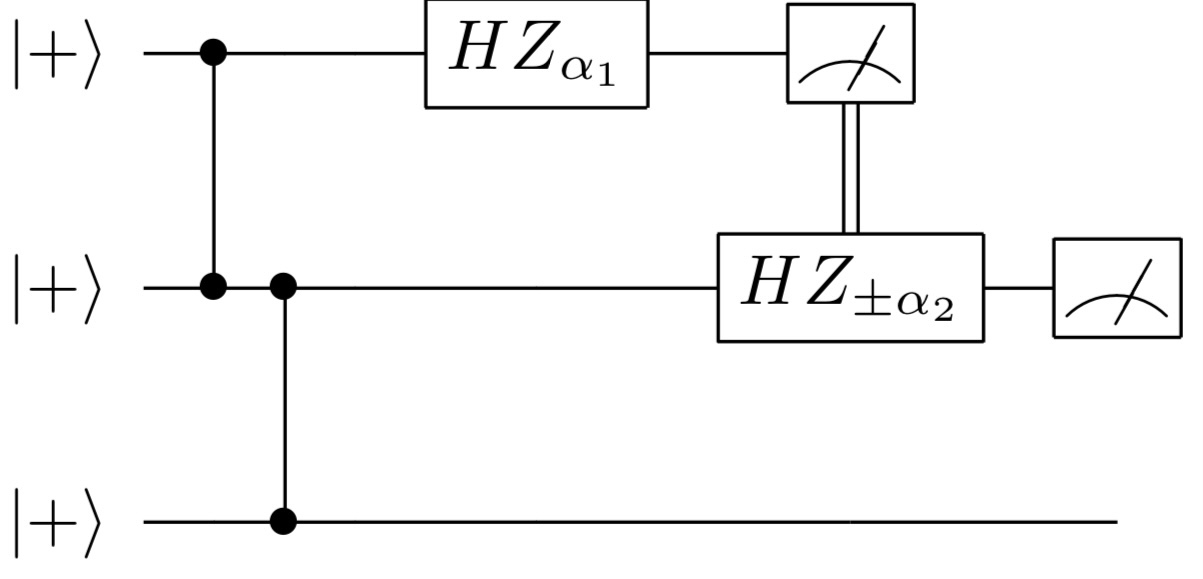Concatenating the single-qubit teleporter allows the input qubit to accumulate single-qubit operations consecutively. Note that the input state acting as a resource for the successive teleporters is a linear graph state.

This provides a means for building up single-qubit operations using a linear graph state. We must also introduce an entangling two-qubit gate to enable universality, which we will choose to be the CZ gate.

Now consider two linear graphs that teleport two input qubits, accumulating single-qubit operations along the way. At some point, we encounter a vertical bridge between these two linear graphs. As the qubits teleport past this bridge, they accumulate a CZ operation between them, as this is identically what the bridge represents.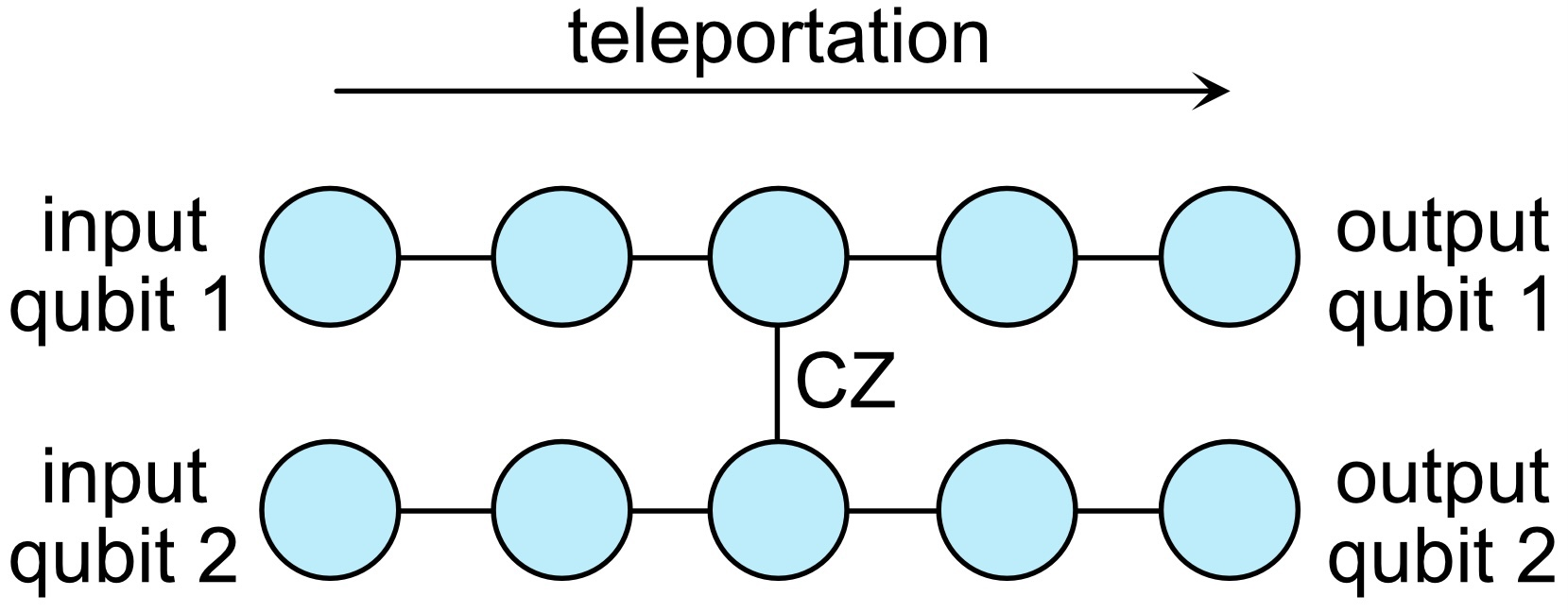A two-qubit graph state computation. The input qubits are successively teleported from left to right as columns are measured out, accumulating single-qubit operations along the way. When the vertical bridge is encountered, they acquire the action of a CZ gate between them, as this is identically what the bridge represents.

Visually, we can see a direct connection between the circuit model and the MBQC model, where rows equate to logical qubits and columns to circuit depth. As columns of qubits are measured out from left to right, qubits are successively teleported from one column to the next, accumulating single-qubit operations along the way. When they encounter vertical bridges, the respective qubits acquire CZ operations, providing everything necessary for a universal quantum computation.

Measurement-based quantum computing could have equally been referred to as teleportation-based quantum computing.

#### Stabiliser representation

Graph states are stabiliser states, meaning that an N-qubit state can be fully specified by N stabilisers, each of which is an N-fold tensor product of Pauli operators up to a factor of \{\pm 1,\pm i\}. A stabiliser is an operator under which the stabilised state is invariant, and the state is uniquely specified as the simultaneous +1 eigenstate of all N stabilisers,

S_i|\psi\rangle = |\psi\rangle,\,\,\forall\, i\in \{1,\dots,N\}.

Graph states have a stabiliser of the form,

S_i = X_i \bigotimes_{j\in n_i} Z_j

associated with every vertex i, where n_i denotes the neighbourhood of i, the set of vertices connected to i by an edge.

The structure of these stabilisers can be understood by noting that the |+\rangle state is stabilised by the X operator, X|+\rangle = |+\rangle. Upon applying a CZ gate between this qubit and a neighbour, the commutation relation,

\mathrm{CZ}_{i,j}\cdot X_i = X_iZ_j\cdot\mathrm{CZ}_{i,j},

implies the X_i stabiliser for qubit i gains an additional Z_j operator for every neighbour j connected by a CZ gate.

Equivalently, stabiliser states are the class of states obtained by evolving computational input states under Clifford operations (i.e. CNOT, CZ, Hadamard, Paulis & phase gates). Note that Clifford circuits map stabiliser states to stabiliser states, which is always classically efficient and insufficient for universal quantum computing [Gottesman (1998)]. However, with the addition of the single-qubit, non-Clifford T gate we obtain a universal gate set.

As an example, consider the following linear graph.

This graph has the stabilisers,

S_1 = X_1 \otimes Z_2
S_2 = Z_1 \otimes X_2 \otimes Z_3
S_3 = Z_2 \otimes X_3

If we were to apply a CZ gate to create a third edge, we would obtain a cyclic graph.

This would then have the stabilisers,

S_1 = X_1 \otimes Z_2 \otimes Z_3
S_2 = Z_1 \otimes X_2 \otimes Z_3
S_3 = Z_1 \otimes Z_2 \otimes X_3

The stabilisers associated with graph states can be represented as binary matrices, also referred to as a tableau representation or generator matrix, comprising an N\times N block representing the location of X operators and another for the location of Z operators,

[\mathbf{X} | \mathbf{Z}].

Noting that rows in this matrix can be arbitrarily permuted, the structure of graph state stabilisers, with exactly one X operator associated with each vertex, implies the X block can be expressed as the identity matrix with appropriate reordering, in which case the Z block corresponds to the adjacency matrix of the graph, noting that the rows and columns of an adjacency matrix capture respective vertex neighbourhoods, the Z operators from each stabiliser,

[\mathbf{X} | \mathbf{Z}] \to[I_N | A_G].

Using the previous example of a three-qubit linear graph, the stabilisers,

S_1 = X_1 \otimes Z_2
S_2 = Z_1 \otimes X_2 \otimes Z_3
S_3 = Z_2 \otimes X_3

can be expressed via the binary matrix,

\left[\begin{array}{ccc|ccc} 1 & 0 & 0 & 0 & 1 & 0 \\ 0 & 1 & 0 & 1 & 0 & 1 \\ 0 & 0 & 1 & 0 & 1 & 0\end{array}\right],

and it can be seen that the Z block corresponds to the expected adjacency matrix for a linear graph.

#### All stabiliser states are locally equivalent to graph states

In addition to graph states being stabiliser states, all stabiliser states are locally equivalent to graph states. An efficient classical algorithm with O(N^3) runtime exists for transforming arbitrary stabiliser states into graph states using local operations [Vijayan et al. (2022)]. Using stabiliser transformation rules in the binary representation, the goal is to diagonalise the X block, achieved using a variant of Gaussian elimination.

The concept is best illustrated by example. Consider the 3-qubit GHZ state, which may be represented using the stabilisers,

S_1 = X_1\otimes X_2\otimes X_3
S_2 = Z_1\otimes Z_2
S_3 = Z_2\otimes Z_3

with the binary matrix representation,

\left[\begin{array}{ccc|ccc} 1 & 1 & 1 & 0 & 0 & 0 \\ 0 & 0 & 0 & 1 & 1 & 0 \\ 0 & 0 & 0 & 0 & 1 & 1\end{array}\right]

Let us first apply Hadamard gates to qubits 2 and 3. Hadamard gates interchange X and Z operators, since HXH=Z and HZH=X, and stabilisers evolve under conjugation. In the binary matrix representation, this swaps the respective columns from the X and Z blocks,

\left[\begin{array}{ccc|ccc} 1 & 0 & 0 & 0 & 1 & 1 \\ 0 & 1 & 0 & 1 & 0 & 0 \\ 0 & 1 & 1 & 0 & 0 & 0\end{array}\right].

Since the product of two stabilisers is also a stabiliser, arbitrarily multiplying them together, equivalent to bitwise XORing the respective matrix rows, does not change the description of the state. Applying S_3\to S_2 S_3, we obtain,

\left[\begin{array}{ccc|ccc} 1 & 0 & 0 & 0 & 1 & 1 \\ 0 & 1 & 0 & 1 & 0 & 0 \\ 0 & 0 & 1 & 1 & 0 & 0\end{array}\right].

Our matrix is now in the required form, and the Z block corresponds to the adjacency matrix of the 3-qubit star graph. In general, an N-qubit star graph is locally equivalent to an N-qubit GHZ state, as is the fully connected graph, K_N.

#### Manipulating graph states

From the definition of a graph state, we know that CZ gates toggle the existence of edges between the vertices upon which they act. Graph states additionally exhibit elegant properties under the action of some gates and measurements, providing valuable tools for directly manipulating the graph structure associated with a state [Hein, Eisert & Briegel (2004), Hein et al. (2006)].

##### Local complementation

One important graph operation that will appear is local complementation (LC), represented by the graph operator \tau_i(G). Local complementation inverts (or complements) the edges in the subgraph induced by the neighbourhood of i.Local complementation about a vertex, denoted \tau_i(G), inverts (or complements) the edges in the subgraph induced by the neighbourhood of i. Here, \tau_1(G) alternates us between the above two graphs. (left) The subgraph induced by the neighbourhood of vertex 1 is the completely disconnected graph with vertices 2, 3 and 4. (right) Upon local complementation, the respective subgraph is the completely connected graph. A further LC operation takes us back to the original graph. Both of these graphs are locally equivalent to the maximally entangled GHZ state.

Applying the square root of the graph stabiliser associated with vertex i,

\sqrt{S_i} = \sqrt{X_i} \bigotimes_{j\in n_i} \sqrt{Z_j},

directly implements the local complementation \tau_i,

\sqrt{S_i}|G\rangle \equiv |\tau_i(G)\rangle,

an isomorphism between the LC graph operator, \tau_i, and the state operator, \sqrt{S_i},

\sqrt{S_i} \cong \tau_i.

Note that \sqrt{S_i} comprises only local operations and hence does not affect entanglement. Nonetheless, we observe that its application generally changes the number of edges in the graph. This leads to the interesting observation that while edges in a graph state represent the application of maximally entangling CZ gates, the amount of entanglement in a graph state is not directly related to the number of edges. Locally equivalent graph states exhibiting identical entanglement structure can significantly differ in their edge count.

The extreme case is seen in the above example of the star and fully connected graphs, related by local complementation around the central vertex. Both of these states are locally equivalent to the maximally-entangled GHZ state, yet one contains the smallest number of edges a connected graph can have, O(N), while the other is maximally connected with O(N^2) edges.

While these are locally equivalent states with identical entanglement structure and computational characteristics, they differ enormously from an architectural implementation perspective. Were these two LC-equivalent states to be physically prepared using CZ gates, the star graph would clearly be preferable owing to its quadratically lower CZ count.

Single-qubit gates are generally far easier to implement than entangling ones, and theoreticians often assume they come for free. From the perspective of minimising CZ gate usage during state preparation, the local complementation orbit of a graph provides an avenue for optimisation.

##### Local complementation orbits

Since local complementation comprises only local operations, all graphs related by LC are locally equivalent. The entire class of graphs related by LC is referred to as the graph’s (local complementation) orbit, whose size, in general, grows exponentially with the number of vertices, |V|.

Note that local complementations about different vertices do not commute in general,

\tau_i\circ\tau_j(G) \neq \tau_j\circ\tau_i(G),\,\,\forall\, G,i,j.

Simultaneously, the LC operation associated with a given vertex is its own inverse, an involution,

\tau_i =\tau_i^{-1},
\tau_i^2 = I.

The LC orbit of a graph admits a non-Abelian group structure, where the LC operators \tau_i act as the group generators, and the graphs in the orbit constitute the elements of the group. The orbit may itself be represented as a graph, the group’s Cayley graph. Here, vertices represent individual graphs in the orbit, and edges, labelled by group generators, relate them via LC operators. Since the LC operators are involutions, the Cayley graph of a graph orbit is undirected.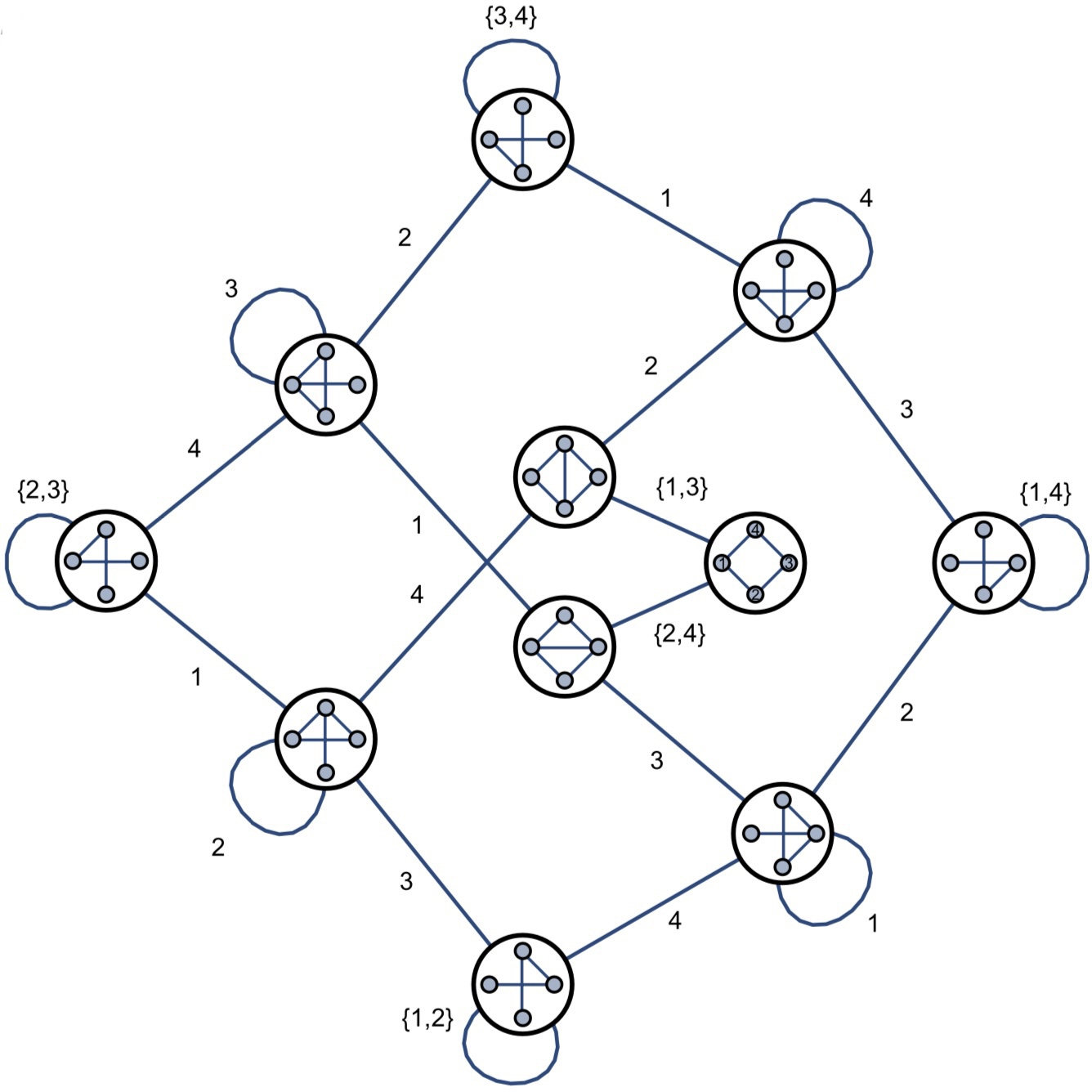The local complementation orbit of the 4-qubit linear graph, represented by its Cayley graph. Vertices represent individual graphs, related by local complementation where an edge exists. Edges are labelled by the indices of the LC operators \tau_i relating graphs. All graphs within an orbit are locally equivalent and exhibit the same entanglement structure, but generally differ in their number of edges. [Figure thanks to Adcock et al. (2020)]

Graph orbits are hard to explore, given their in-general exponential scaling and the complexity of navigating them. Finding the LC-equivalence between two graphs is NP-complete in general, while the problem of counting the orbits of a graph is in general #P-complete [Dahlberg, Helsen & Wehner (2019); Adcock et al. (2020)].

A sequence of LC operations,

\tau_{\vec{u}}(G) = \tau_{u_{|u|}} \circ \dots \circ \tau_{u_1}(G)

may be represented by an ordered (since LC operators are non-commutative) list of vertex labels where complementations took place,

\vec{u} = \{u_1,\dots,u_{|u|}\},\,\,u_i\in V\,\,\forall\,i,

which may be of any length and have repeat entries.

A graph G’ is said to be a vertex-minor of G if it can be reached from G via a sequence of local complementations, \vec{u}, and vertex deletions, for which we use the notation,

G’<G.

The vertex-minor problem is the decision problem of finding a sequence of local complementations, \vec{u}, such that \tau_{\vec{u}}(G) = G’, up to vertex deletions, which may always be performed at the end.

This problem is known to be NP-complete in general, requiring exponential classical (and quantum) runtime. While complexity proofs are onerous, the intuition behind the NP-completeness of the vertex-minor problem can be seen by framing it as a satisfiability problem.

Defining the polynomial-time function,

f(\vec{u},G,G’)\to \begin{cases} 1, & \tau_{\vec{u}}(G) = G’ \\ 0, & \tau_{\vec{u}}(G) \neq G’ \end{cases}

solving vertex-minor is now equivalent to finding a satisfying input string, \vec{u}, such that f(\vec{u},G,G’)=1. Unstructured satisfiability (or SAT) problems of this form are NP-complete in general, noting there is more nuance than this as this is not an unstructured satisfiability problem.

Counting the orbits of the N-qubit GHZ state is definitely not #P-complete, as there are always exactly two. It therefore exhibits O(1) time-complexity, where 1=2.

##### Pauli measurements

Up to measurement-outcome-dependent local corrections, Pauli measurements implement simple graph transformation rules as follows:

• Pauli-Z: Delete vertex i from G,

G\to G-i.
• Pauli-Y: Locally complement the neighbourhood of i and delete vertex i,

G\to \tau_i(G)-i.
• Pauli-X: For any qubit b neighbouring i, locally complement b, apply rule for Y, then locally complement b again,

G\to \tau_b(\tau_i \circ \tau_b(G)-i).The effect of Pauli X, Y and Z measurements on the red qubit in the linear graph state shown at the top. In all cases, the measured qubit is detached from the graph by removing all its connecting edges. The choice of measurement basis additionally imposes an update rule on the remaining edge set.

Since Pauli measurements induce simple graph transformation rules, this immediately implies that graph states combined with Pauli measurements are classically efficient to simulate; simultaneously, Pauli measurements alone are insufficient for universal MBQC, which cannot be efficiently classically simulated. Furthermore, any local Clifford operation may be expressed in terms of efficient graph transformation rules [Van den Nest, Dehaene & De Moor (2004)]. This observation is analogous to the Gottesman-Knill theorem [Gottesman (1998)], that stabiliser states in combination with Clifford evolution and Pauli measurements are classically efficient to simulate, recalling that all stabiliser states are locally equivalent to graph states.

#### Graph surgery

These measurement properties facilitate convenient surgical graph operations. Suppose we became aware that we lost a qubit from a graph state. Rather than discard the entire state and start from scratch, we can measure out the neighbouring qubits in the Z-basis, thereby detaching the lost qubit from the graph, after which the damaged section can be reconstructed by replacing the measured and lost qubits with fresh ones and reconnecting them using CZ gates.If a qubit is lost (red) from a graph state, discarding the entire state and preparing from scratch is unnecessary. Instead, we can measure out its neighbouring qubits (orange) in the Z basis (left), replace the lost and measured qubits with fresh |+\rangle states (green), and apply the necessary CZ gates to reconstruct the lost edges (right). Edges outside the defect’s neighbourhood are unaffected (blue vertices on the left).

Pauli measurements also provide the tools for contracting larger graphs with redundant qubits down to ones with the required substructure, thereby etching the structure into the graph. Any subgraph of G induced by vertex set S, G[S], may be obtained by measuring out all vertices V(G-S) in the Z basis, while Y measurements may be employed to perform contractions. This provides an elegant means for arguing that lattices of various topologies are universal for MBQC — if a lattice can be reduced to substructures reflecting arbitrary quantum circuits, it can be considered a substrate for universal MBQC.Reducing a lattice graph to one with two horizontal linear chains connected by a single vertical bridge, analogous to the earlier graph for simulating a circuit comprising single-qubit operations and a CZ gate.

#### Graph state compilation

Quantum algorithms are often designed in the circuit model. While etching circuits into a corresponding graph structure works by equivalence, it doesn’t exploit the more general graph-theoretic structure of graph states, rather imposing an existing way of thinking onto a different paradigm in a highly constrained way.

A more direct and resource-efficient approach for the compilation of quantum circuits into graph states was presented by Vijayan et al. (2022), known as algorithm-specific graph states, distinguishing them from universal ones like lattices. Conceptually, the approach is to structure quantum algorithms into a form where all Clifford circuitry — the bulk of most quantum algorithms — acts on the computational input state, thereby preparing a stabiliser state, while non-Clifford gates are performed at the end and may be absorbed into non-Clifford measurements.

Considering a universal gate set comprising Clifford and T gates, it is only the non-Clifford, single-qubit T gates that present an obstacle to graph state representation. One well-known technique for converting Clifford + T circuits into Clifford-only circuits is using magic state injection. Here, performing single-qubit teleportation using a,

|A\rangle=T|+\rangle=(|0\rangle+e^{i\pi/4}|1\rangle)/\sqrt{2}

resource state, known as a magic state, implements quantum gate teleportation of a T gate. Since the teleportation circuit itself is Clifford-only, this allows any circuit to be expressed in a form comprising only Clifford operations, where some inputs are non-stabiliser states.

The T gate teleportation protocol can also be inverted, relying on measurement of the non-Clifford

A(\pi/4)=T^\dag X T

observable instead of magic states. This inverted form allows Clifford + T circuits to be expressed as stabiliser states followed by non-Clifford measurements.T gate teleportation using the inverse-ICM formalism. This circuit teleports the action of a T gate onto |\psi\rangle. Unlike the conventional approach of magic state injection, which relies on the non-stabiliser resource state |A\rangle=T|+\rangle=(|0\rangle+e^{i\pi/4}|1\rangle)/\sqrt{2}, the inverted model relies only on a |0\rangle resource state, absorbing the T gate into measurement of the non-Clifford A(\pi/4)=T^\dag X T observable. Substituting this sub-circuit in place of all T gates within a Clifford + T circuit converts it to a form comprising stabiliser state preparation followed by non-Clifford measurements, equivalently a graph state followed by non-Clifford measurements.

Since stabiliser states are locally equivalent to graph states, this decomposition allows universal quantum circuits to be directly compiled to graph states, where computation proceeds via non-Clifford measurements.

Algorithm-specific graph states capture the entanglement structure of algorithms much more naturally than an etched circuit would. However, the resultant graphs are not unique, as their orbits are computationally equivalent.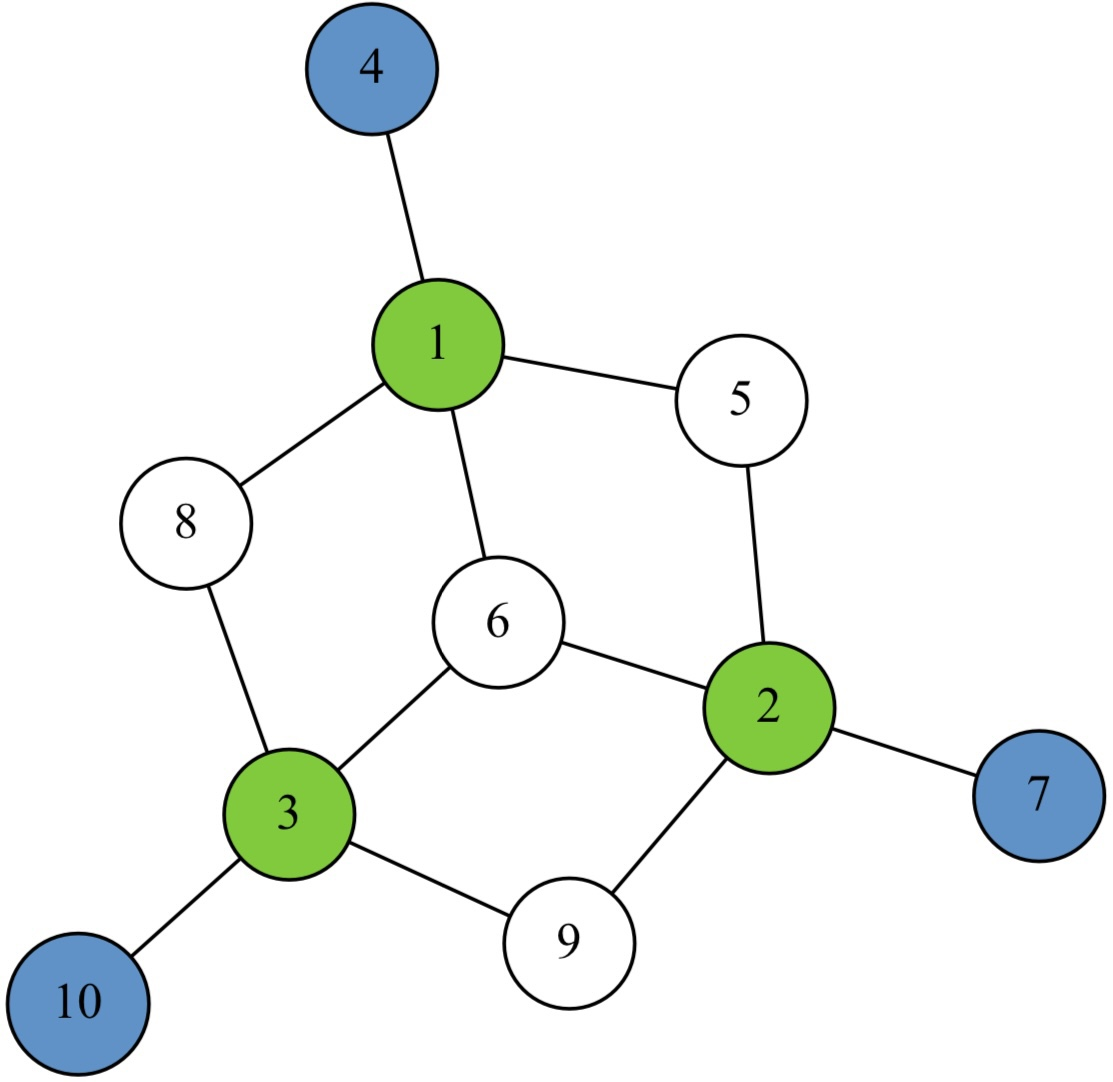Compilation of a Toffoli gate acting on the |+,+,0\rangle input to a graph state, where input and output qubits are shown in green and blue, and all qubits except the outputs are measured in the A(\pm\pi/4) basis. [Figure thanks to Vijayan et al. (2022)]

Graph optimisation therefore becomes an important next stage in the compilation pipeline. The optimal graph for implementing a given algorithm has many variables and is subject to architectural constraints, but identifying them generally yields computationally complex optimisation problems associated with the complexity of exploring graph orbits.

#### Preparing graph states

The commutativity of CZ gates implies they are order-independent. In the context of graph states, this means edges needn’t be built up sequentially but can be parallelised, and divide-and-conquer strategies may be employed to build up large graph states from smaller subgraphs that may be prepared independently and in parallel.

This becomes especially useful when entangling gates are non-deterministic, as is often the case in many physical architectures and necessarily the case when dealing with photonic implementation.

Consider a linear graph state with N qubits. One might think that preparing this state requires successfully performing N-1 CZ gates in succession, meaning that if the gates are non-deterministic with success probability p_\mathrm{gate}, the overall state preparation probability would be p_\mathrm{gate}^{N-1}, which is exponentially decreasing and therefore inefficient.

However, this is not the correct intuition for graph states, which are generally not maximally entangled and from the Pauli measurement properties, enable a state to be rescued via surgical operations when local defects occur.

Rather than building the linear graph in a single shot, let us consider the following scenario, taken from Rohde & Barrett (2007). We have a main linear graph of length N and a resource of small linear graphs of length M, which are prepared offline. We are assuming the availability of quantum memory, or qubits with inherently long decoherence times, to enable this protocol.

We will employ a CZ gate that succeeds with probability p_\mathrm{gate}, and upon failure, destroys the qubits it acts upon, effectively tracing them out of the system.

Upon applying the CZ gate between the two graphs of length N and M, with probability p_\mathrm{gate}, the main graph grows in length to N+M. Upon failure, with probability 1-p_\mathrm{gate}, the end qubit is destroyed, and we recover the remainder of the graph by measuring out its neighbour in the Z basis, shrinking it in length to N-2.

Applying this process repeatedly, the length of our main graph proceeds as a random walk, probabilistically growing or shrinking. The figure of merit is the average length by which the graph grows or contracts. In our case, this can easily be calculated to be,

\langle\Delta\rangle = p_\mathrm{gate}M - 2(1-p_\mathrm{gate}).

If the graph is to grow on average, we require \langle\Delta\rangle > 0, which implies,

M>2(1-p_\mathrm{gate})/p_\mathrm{gate}.

A CZ gate operating with 50% probability would only require M=3 resource states to ensure growth on average.

So long as the resource states we employ are at least length M, our main graph is guaranteed to grow on average, with length growing linearly in the number of iterations t,

\langle N(t)\rangle = N(0) + \langle\Delta\rangle\cdot t.When bonding a primary linear graph state of length N with a smaller resource state of length M using a non-deterministic CZ gate with success probability p_\mathrm{gate}, the primary graph either: grows in length to N+M with probability p_\mathrm{gate}; or, after recovery using a Z measurement to detach the destroyed qubit, shrinks in length to N-2 with probability 1-p_\mathrm{gate}. Repeating this process, the primary graph’s length evolves as a random walk. If the resource states have length M>2(1-p_\mathrm{gate})/p_\mathrm{gate}, the primary graph will grow on average, increasing linearly with time.

While the above argument is for linear graph states, linear graphs alone are not computationally useful. For MBQC, we require at least a two-dimensional topology, such as a lattice. It was first shown by Nielsen (2004) how graph structure can be exploited to enable efficient preparation of arbitrarily large lattice graph states using non-deterministic gates by introducing the concept of micro-clusters. Micro-clusters are small subgraphs of a larger graph state with multiple dangling nodes, each providing an independent bonding opportunity. Micro-clusters, therefore, provide redundancy in bonding attempts should they sometimes fail.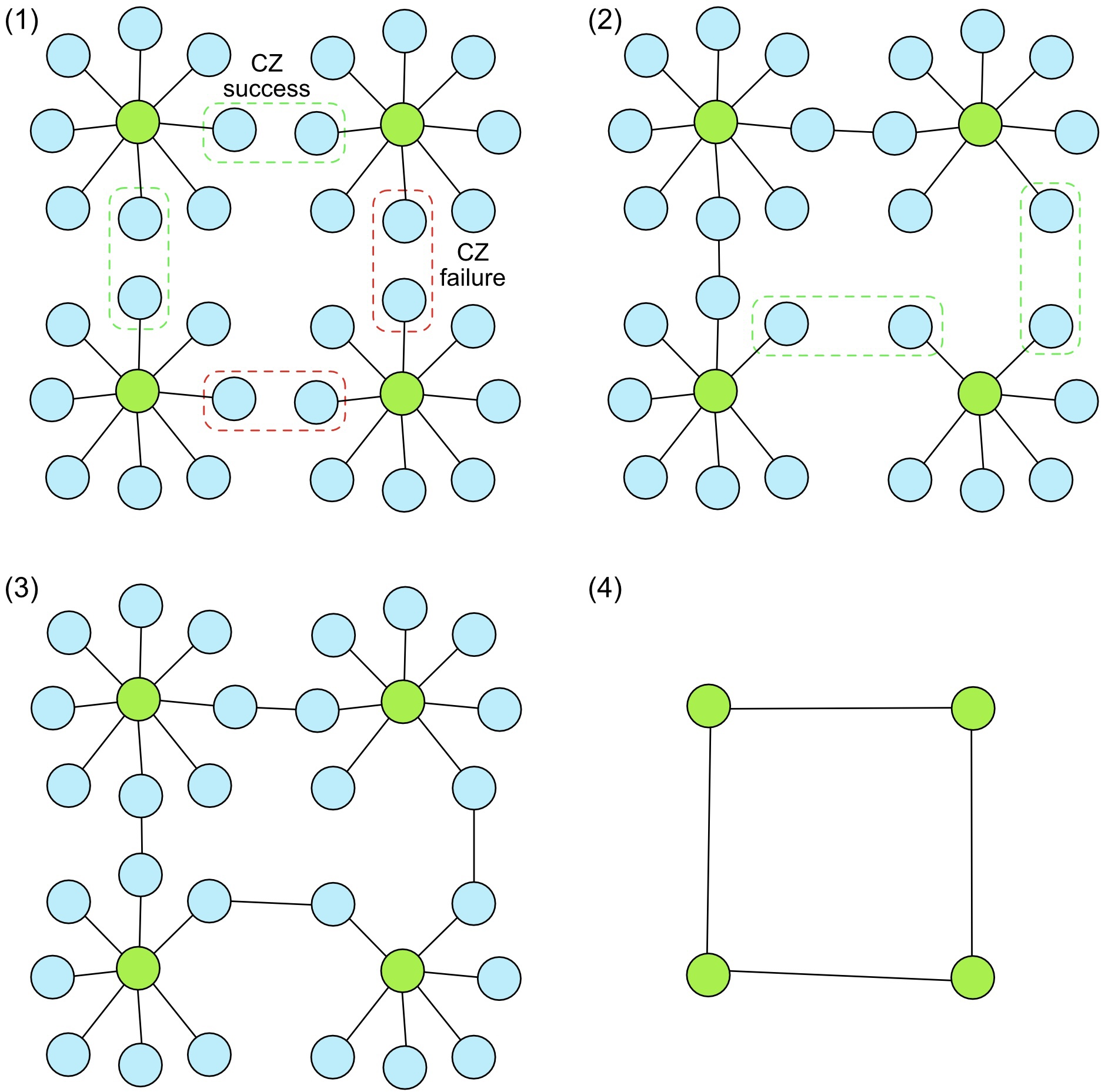Preparing a 2\times 2 lattice graph state using four micro-clusters. Each micro-cluster comprises a central vertex (green), to belong to the final lattice graph. The dangling bonds (blue) provide multiple opportunities to attempt bonding with neighbouring micro-clusters. If a CZ bonding attempt fails (red), the respective dangling bonds are removed. Upon success (green), the respective micro-clusters are connected, albeit with some intermittent vertices. Once bonds exist in each direction, redundant vertices are measured out in the Pauli Y basis, contracting the graph down to the desired 2\times 2 lattice.

How large do micro-clusters need to be? The probability of a micro-cluster failing to bond with another drops exponentially with the number of available attempts, M,

p_\mathrm{fail} = (1-p_\mathrm{gate})^M,

which implies the number of available bonds must scale as,

M>\frac{\log(p_\mathrm{fail})}{\log(1-p_\mathrm{gate})},

which is only logarithmic and highly efficient. Achieving 99% bonding probability using gates with 50% success probability requires only M=7 available bonds.

This observation affords enormous resource savings in the context of linear optics quantum computing (LOQC), where micro-clusters were first introduced. Resource overheads associated with the non-determinism of entangling gates in LOQC using the initial circuit-based construction described by Knill, Laflamme & Milburn (2001) (aka KLM) were ‘efficient’ in the computer scientist’s sense of exhibiting polynomial scaling but not in a practical sense as the polynomials were unpleasant ones.

#### Percolation theoryAn edge-percolated lattice for p_\mathrm{delete}=0.7, 0.4 and 0.1. Asking whether a route exists across the graph, there will exist a percolation threshold probability, p_c, above which route existence is unlikely, below which it is likely. The middle figure corresponds to the associated threshold probability, where on average, a path is likely to exist across the graph, but if the deletion rate were increased would quickly lose connectivity and break into disconnected islands, as per the left figure. [Figure thanks to Browne et al. (2008)]

Percolation theory studies the connectedness of percolated graphs (ones with random defects) as a function of defect rate, p_\mathrm{delete}. Percolations could apply to either edges or vertices, and the measure of connectedness could be defined in many ways, commonly whether routes exist spanning the graph.

Taking an edge- or vertex-percolated lattice in the limit of large lattice dimension, L, and considering the probability of connectedness as a function of percolation probability, p_\mathrm{delete}, percolation theory predicts phase-transitions in graph connectivity at some percolation threshold, p_c.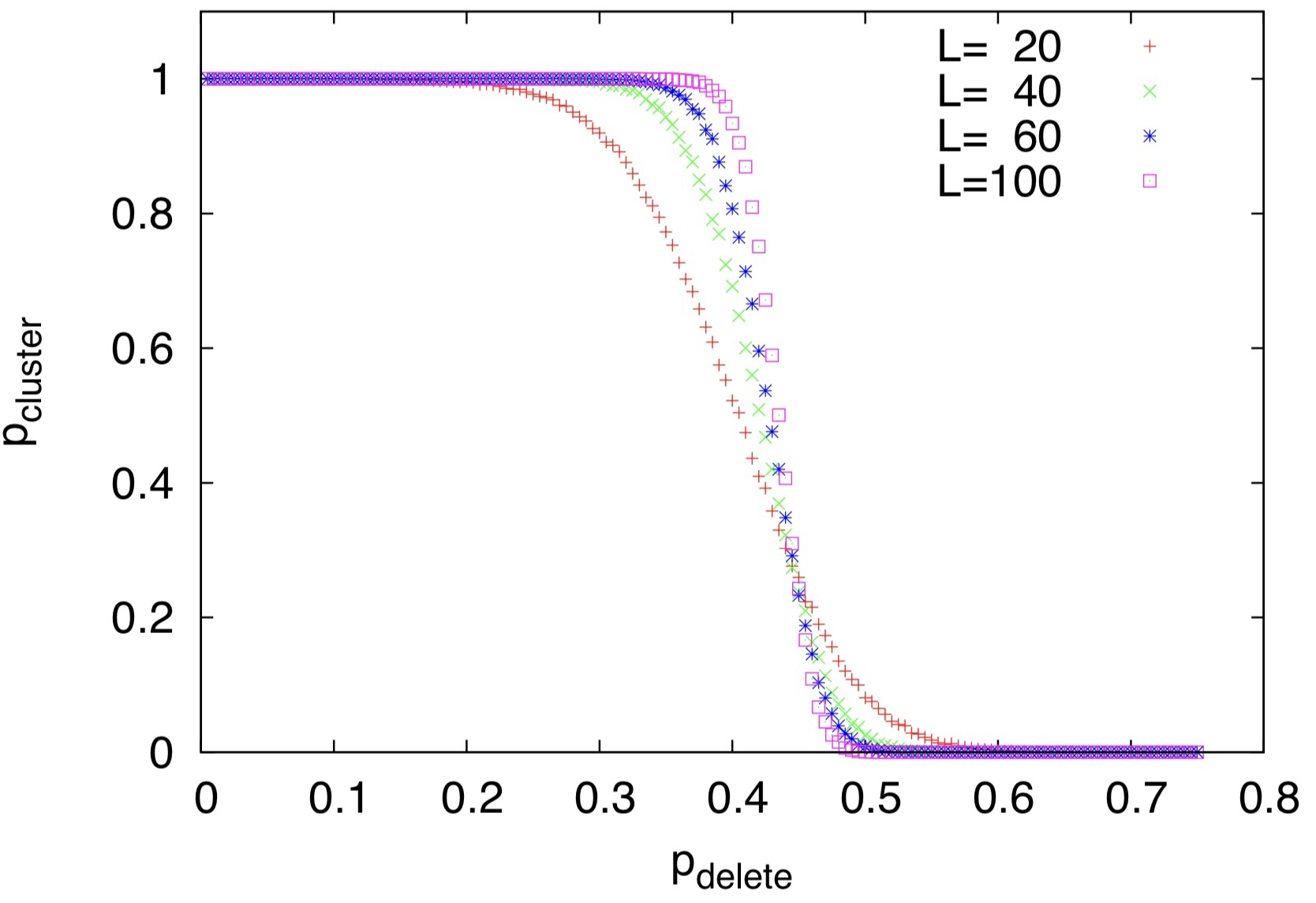Probability of the existence of a spanning cluster across a lattice of dimension L against edge deletion probability p_\mathrm{delete}. In the limit of infinite lattice dimension, L\to\infty, the curve approaches a step function, marking the percolation threshold, p_c, of a phase-transition in graph connectivity. [Figure thanks to Wei, Affleck & Raussendorf (2012)]

Consider a lattice of micro-clusters. As a function of their size and gate success probability, there exists an associated probability of edge deletion in the subsequent reduced substrate graph.

The relationship between lattice dimension and computational power implies one between percolation probability and computational power [Browne et al. (2008); Pant et al. (2019); Wei, Affleck & Raussendorf (2012)]. Since the likelihood of finding paths through a graph is a function of the percolation rate, so is the expected density, hence dimension, of a lattice reduced from the available paths.

A percolated lattice graph state with missing qubits (i.e. vertex percolations — one could likewise consider edge percolations), where squares are qubits, yellow if present.

A route-finding algorithm finds sets of edge-disjoint left-to-right (red) and top-to-bottom (green) paths. The path density is a function of the percolation rate.

The path set is reduced to a regularised substrate, here an alternating bridge decomposition, a resource for universal MBQC.

All qubits not belonging to our path-set are measured out in the Z basis, while paths are contracted using Y measurements, reducing the graph to a regularised substrate of some dimension, which directly relates to its computational power.

This yields a relationship between computational power and percolation probability, where we observe computational phase-transitions for large graph dimension, L\to\infty. Above threshold, p_\mathrm{delete}>p_c, we rapidly lose computational power as the graph disconnects into islands and spanning paths cease to exist. The percolation threshold is an important parameter for engineering scalable architectures using this approach.

Since the dimension of the residual lattice is a function of the percolation rate, in turn a function of micro-cluster size and gate success probability, there exists a trade-off between the resources invested into micro-cluster size and computational return, a point of optimisation from an engineering perspective.

#### Entangling operations

Although by definition, the edges in graph states represent the action of CZ gates, these are not the only gates capable of growing graph states. Depending on the physical implementation, various other approaches to creating graph edges exist.

In photonic quantum computing, fusion gates [Browne & Rudolph (2005)] can be used to create connections in graphs. Fusion gates are partial Bell measurements, implemented using polarising beamsplitters (see my previous post, “How do photonic Bell measurements work?”). While fusion gates create new edges in a graph state, they are also destructive, meaning they destroy the two qubits they operate on. This implies some resource overhead, although this overhead is far more favourable than that imposed by optical CZ gates, which are highly complex and resource intensive to implement.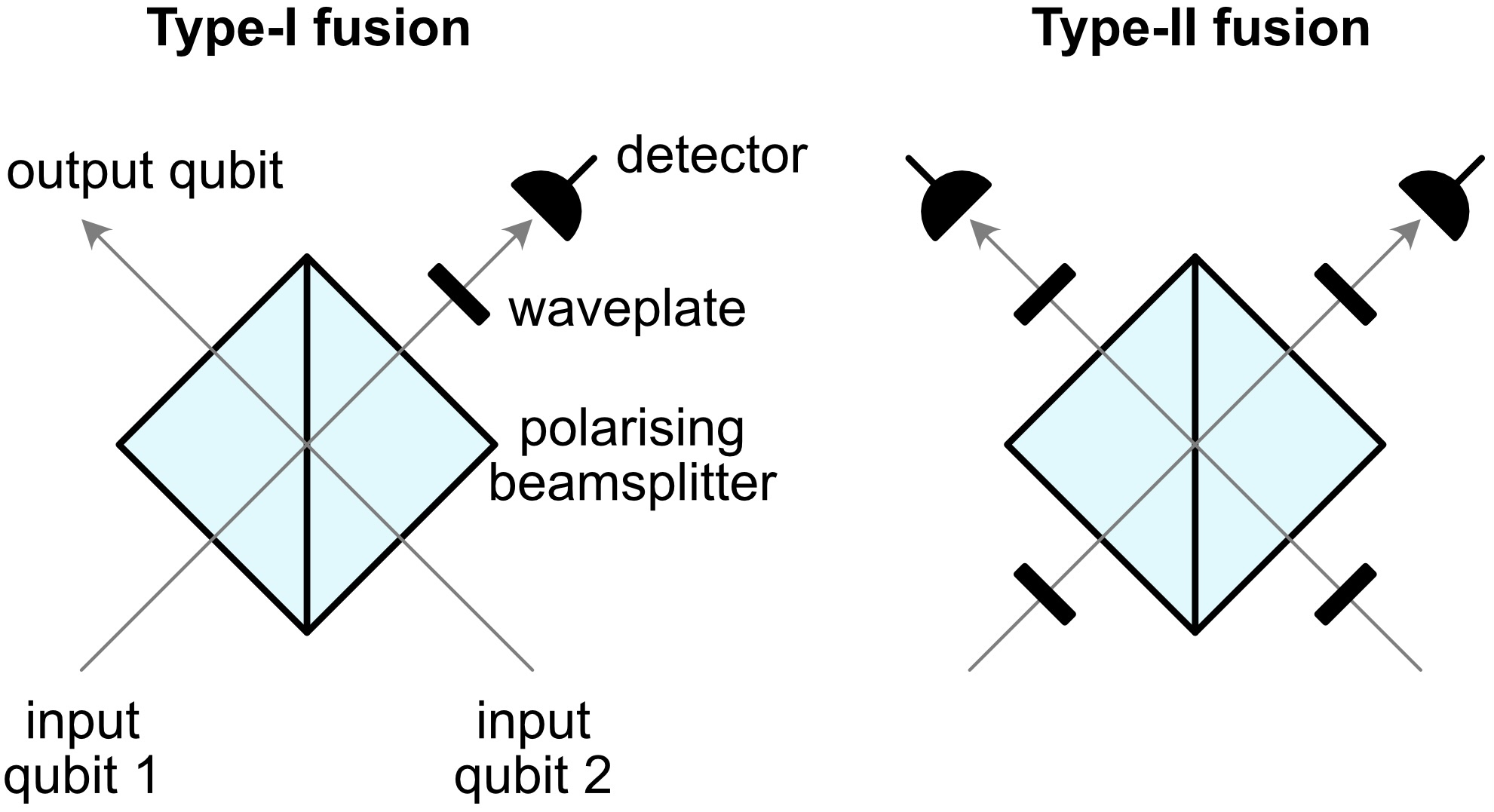The type-I and -II fusion gates enable the creation of edges in graph states comprising polarisation-encoded qubits. Unlike CZ gates, fusion gates are destructive, consuming one (for type-I) or both (for type-II) of the qubits they operate on, creating edges within their neighbourhood that differ from the CZ edge-creation rule, but are nonetheless sufficient for engineering arbitrarily large graph states. These gates are non-deterministic with a 50% success probability.

In atomic systems with suitable level structure, where energy levels couple to optical modes, a beamsplitter followed by photo-detection implements which-path erasure on photons emitted via relaxation processes, projecting the two atoms into a symmetric superposition of one being excited state and the other relaxed, equivalent to a Bell projection.

#### Error propagation

As with quantum circuits, graph states are subject to various error models. How do these propagate through graph states?

Since quantum information flows through graph states via teleportation, so will errors. Consider the single-qubit teleportation circuit, where the local correction applied to the second qubit depends on the measurement outcome of the first. If a Pauli error was introduced onto the first qubit, flipping its measurement outcome, m, this would subsequently manifest itself as a flipped local correction on the second qubit, thereby propagating the error.

In general, as qubits are measured out of a graph state, errors acting upon them are teleported onto neighbouring qubits and accumulate. In the context of micro-cluster-based approaches for tolerating gate failure, the dangling bonds facilitating multiple bonding attempts, which must subsequently be removed, imposes a trade-off between errors. While more dangling bonds implies greater tolerance against gate failure, it simultaneously implies lower tolerance against Pauli errors, which accumulate whenever a redundant bond is removed via measurement.

It is desirable to avoid unnecessarily preparing and subsequently measuring out redundant qubits, and the overall design of a measurement-based architecture must carefully evaluate the inherent tradeoffs between different error types.

Although lattice graphs are universal for MBQC, as arbitrary circuit structures can be etched into them, in addition to being wasteful, this results in unnecessary accumulation of errors.

#### Quantum error correction

Graph states are not inherently error-protected, and quantum error correction (QEC) is required as with any other model of quantum computation. Thankfully, this does not require abandoning the inherent elegance of graph states as there are graph-native QEC codes. In particular, topological codes naturally lend themselves to graph-based implementation [Raussendorf, Harrington & Goyal (2006)].

An example is the surface code [Kitaev (2003)], defined relative to a square lattice graph. Although the way surface code stabilisers are defined is distinct from graph states, a direct mapping exists between them under appropriate graph transformations [Bravyi & Raussendorf (2007)].The surface code is defined relative to a graph, albeit with a different convention in defining stabilisers. Qubits are associated with graph edges, and two types of stabilisers define the state: every square (blue) is associated with a plaquette operator acting on its four constituent qubits, S_\square = X^{\otimes 4}; and, every star (red) with a star operator, S_+ = Z^{\otimes 4}. The property that products of stabilisers are also stabilisers lends itself to elegant geometric interpretations. For example, taking the product of a set of neighbouring S_\square operators implies a chain of X operators around the boundary of a closed region of the surface is also a stabiliser.

Such graph-based QEC codes connect us with the field of topology, where the manifestation of errors and implementation of logical operations may be defined in terms of topological invariants — properties that remain invariant under continuous deformation, from which they inherit their robustness.

For example, continuously deforming a non-trivial loop around a torus (see figure below) preserves its topological characterisation. Associating logical operators with operator strings around a loop bestows redundancy in how the logical operator can be applied. Should defects rule out a particular path, another topologically equivalent one can be chosen, allowing defects to be bypassed.Imposing periodic boundary conditions on the surface code yields the toric code, defined over the surface of a torus. Here logical X (blue) and Z (red) operators are associated with chains of respective Pauli operators acting on the qubits around topologically distinct closed loops. The topological characterisation of these loops is invariant under continuous deformation, as are the logical operations associated with them. Note that the red and blue chains are topologically distinct and cannot be continuously deformed into one another. In contrast, the two blue loops are topologically equivalent and correspond to the same logical operator. Conceptually, the error tolerance of the toric code stems from the robustness of these topological invariants against defects, scaling with surface dimension.

#### Fusion-based quantum computing

Fusion-based quantum computing [Bartolucci et al. (2021)] is a scalable, fault-tolerant, photonic architecture (although also applicable to other physical architectures), integrating many of the concepts we have discussed. This scheme admits more elaborate resource states with small QEC codes built into them, such that some qubits act as parity checks when measured.(a) A hexagonal resource state can act as a unit cell in a three-dimensional fusion network. (b) A graph substitution rule allows each qubit to be encoded into a (2,2)-Shor code. (c) An error-protected hexagonal resource state, obtained by substituting (b) into (a). [Figure thanks to Bartolucci et al. (2021)]

Fusion gates (non-deterministic, destructive Bell measurements), implemented using polarising beamsplitters (discussed earlier), enable fusion between unit cells of encoded resource states into a three-dimensional fusion network with random defects associated with unsuccessful fusions.

Fusion measurement outcomes reveal a percolated three-dimensional lattice with average case connectivity sufficient to post-select a substructure supporting a scalable topological code in addition to the syndrome outcomes for the associated code.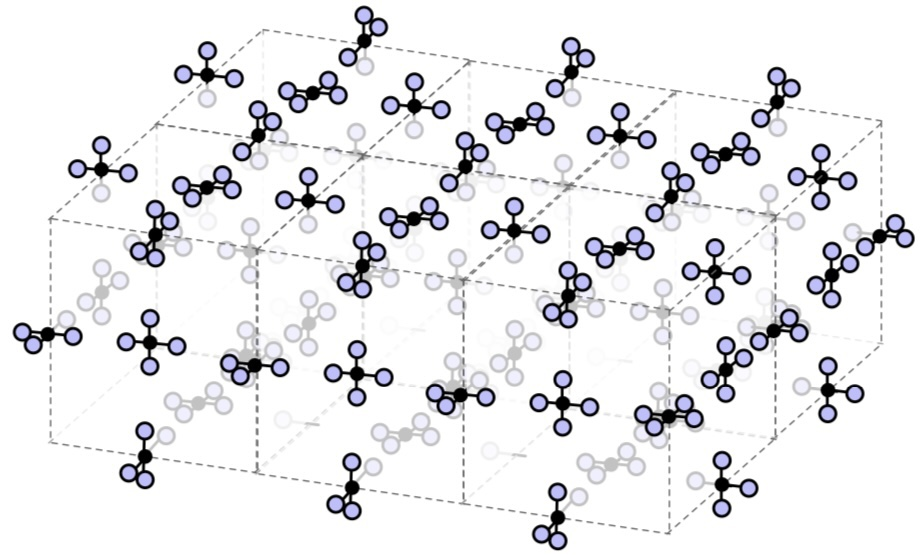A three-dimensional fusion network using 4-star resource states. Fusion operations are non-deterministic, and the fused structure will be percolated. With sufficient average-case connectivity, substructures can be post-selected that encode a measurement-based quantum computation into a topological code whose syndrome measurements are revealed by fusion outcomes. [Figure thanks to Bartolucci et al. (2021)]

#### Quantum communications networks

The goal of quantum communications networks is usually to distribute long-range Bell pairs, equivalently two-qubit graph states. Bell pairs act as a universal resource for quantum communication as they enable quantum state teleportation.

Since long-range quantum communication is generally very lossy, with attenuation increasing exponentially with distance, quantum repeater networks (or entanglement distribution networks) use divide-and-conquer techniques to subdivide long-range links into multiple short-range ones, which are iteratively merged using entanglement swapping, enabling the exponential scaling of loss with distance to be overcome and replaced with polynomial scaling.An efficient divide-and-conquer approach for long-range entanglement distribution in the presence of lossy channels. Execution flows from bottom to top; blue nodes represent Bell pairs, and ones with child nodes are prepared by entanglement swapping them, reducing two shorter-range Bell pairs into one longer-range one. It is assumed that quantum memory is available, such that a parent node can await both its children and branches can execute in parallel. Runtime is exponential in the depth of the tree since the root node, representing the final long-range Bell pair, requires all child nodes to succeed. However, since the binary tree execution order has only logarithmic depth in the number of initial short-range Bell pairs, this directly counters the exponential, resulting in net polynomial scaling in both time and Bell pair consumption.

This can also be conceptualised in terms of graph states. Based upon the same intuition for efficiently growing arbitrarily large graph states using non-deterministic gates, graph states can similarly be employed for efficient entanglement distribution in the presence of lossy channels, allowing the exponential attenuation of single-shot transmission to be overcome and replaced with efficient polynomial scaling.

Our graph state tools for dealing with gate failure (equivalently loss) can be adapted for this purpose. Using micro-cluster-type concepts, redundant bonds counter channel loss by facilitating multiple bonding attempts, where the failure of a single attempt does not compromise the remaining state.The complete-like micro-cluster graph, |\bar{G}_c^m\rangle, here with m=4. (blue) The complete graph, K_{2m}, has an edge between every pair of the 2m vertices. Deleting all but two leaves us with a K_2 graph, a Bell pair between the remaining two qubits. The measurements determining which two are chosen can be made at any stage and deferred. Transmitting the left and right halves of the dangling bonds (yellow) to different parties via lossy channels enables m transmission attempts in each direction. If at least one qubit from each half reaches its destination, appropriate measurements on the K_{2m} subgraph retrospectively routes them together. The likelihood of complete failure decreases exponentially with m.

Applying this iteratively, the goal is to engineer a distributed graph state, which can subsequently be reduced to a long-range Bell pair upon measuring out redundant, intermittent qubits.A pure graph state-based quantum repeater for long-range entanglement distribution over lossy channels [Azuma, Tamaki & Lo (2015)]. Source nodes, C^s, distribute routing substrate graphs (grey, as per previous figure) with m-fold redundancy (here m=3), |\bar{G}_c^m\rangle, which receiver nodes, C^r, fuse together using probabilistic Bell measurements. The m-fold bonding redundancy facilitated by the |\bar{G}_c^m\rangle states enables non-determinism associated with channel loss and gate failure to be asymptotically suppressed with m, allowing efficient preparation of a distributed graph state, which can subsequently be reduced to a long-range Bell pair. This is a direct graph-theoretic generalisation of an ordinary Bell pair entanglement swapping network, which this reduces to for m=1. [Figure thanks to Azuma, Tamaki & Lo (2015)]

In addition to their utility in efficiently overcoming loss in quantum repeater networks, distributed graph states act as a versatile resource for entanglement distribution. Assuming nodes in the network are cooperative and classically communicating, we have the tools to etch out subgraphs (using Z measurements) and contract them down to involve only the desired parties (using X and Y measurements).

Of particular interest are Bell pairs. Our graph transformation rules for Pauli measurements guarantee that any connected graph may be reduced to a Bell pair between any pair of nodes.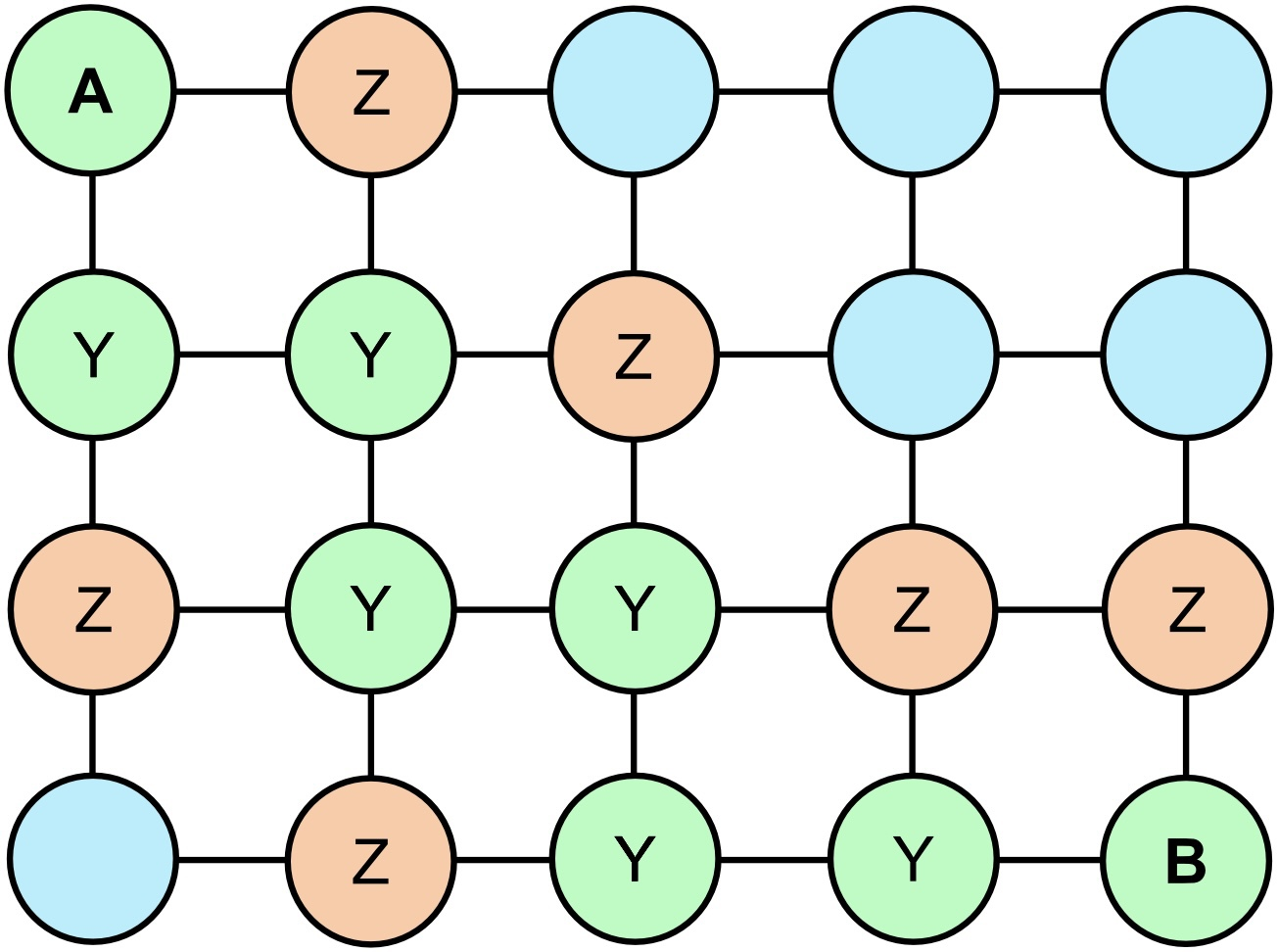Entanglement routing by reducing a large, distributed graph state to a Bell pair between Alice (A) and Bob (B). First, we identify a path between Alice and Bob. All nodes neighbouring the path measure their qubits in the Z basis, detaching the path from the remainder of the graph. All nodes belonging to the path (except Alice and Bob) measure their qubits in the Y basis, contracting it to a two-qubit graph state (i.e. a Bell pair) between Alice and Bob.

Other states of interest might also be available depending on the initial graph’s topology. GHZ states are of interest as they are maximally entangled states that facilitate various multiparty quantum protocols such as open-destination quantum state teleportation and quantum anonymous broadcasting [Christandl & Wehner (2005)]. These can be realised by etching out star graphs. In the special case of 3-qubit GHZ states, any connected 3-qubit graph state, of which there are only two (star and fully connected), is locally equivalent to a GHZ state, and any connected graph with at least three qubits can necessarily be reduced to these using Pauli measurements.

However, we are not limited to measurements when manipulating distributed graph states. We can also play local complementation games, which can be exploited in graph-state-based entanglement routing [Hahn, Pappa & Eisert (2019)].

#### Distributed quantum computing

In a futuristic scenario where large-scale quantum computers and quantum communications networks are readily available, there is a strong incentive to unify geographically dispersed quantum computational resources. Given that the power of quantum computers can, depending on the application, grow exponentially with their number of logical qubits, unifying quantum computers to act as one effectively multiplies their power, which would only accumulate additively in the absence of unification, as per classical computers.

Graph states provide an elegant framework for conceptualising how to architecturally achieve this unification.

The dimensions of the underlying lattice graph dictate the size and power of a MBQC. In the earlier example, the width of the lattice equated with circuit depth and height with the number of logical qubits involved in the computation.

Suppose two independent quantum computers individually had the capacity for N\times N lattice graphs. Rather than use them separately, with quantum communication at our disposal, enabling the creation of long-range links, our two units could stitch their lattices together in a patchwork manner, providing a distributed 2N\times N lattice, affording twice as many logical qubits or twice the circuit depth, an idea that logically generalises to any topology or number of nodes.Unifying two geographically dispersed graph states into a larger distributed graph state using long-range Bell pairs provided by an entanglement distribution network. The increased dimension of the unified graph facilitates a larger MBQC in terms of circuit depth or logical qubit count, depending on orientation. The idea logically generalises in an obvious way, enabling an arbitrarily large distributed graph to be prepared by patchwork. [Figure thanks to Leone et al. (2021)]

#### Conclusion

Graph states are often equated with measurement-based quantum computing and seen merely as a different way of achieving the same thing. However, graph states provide far more than a direct equivalence with other models of quantum computation, like the circuit model. They provide an alternate framework for conceptualising the flow and interaction of quantum information, enabling unique insights that would not come naturally from the confines of other models. These insights have been of enormous value beyond computation, finding widespread utility in other quantum information processing protocols.

The geometric abstractions graph states provide have facilitated significant advances in solving practical problems, without which a quantum future would be far less likely. In photonic quantum computing, for example, implementation via the circuit model imposes formidable — effectively prohibitive — resource overheads, for which the graph state model affords natural solutions.

Graph states have enabled quantum information processing to be united with otherwise disparate fields of mathematics, providing direct connections with graph theory, percolation theory and topology. Graph theory provides powerful tools for conceptualising the flow of quantum information and associating quantum operations with graph transformations. The connection with the field of percolation theory offers insight into the relationship between errors and quantum computational power. Graph theoretical approaches towards quantum error correction intimately connect with the field of topology, in which codes and operations can be abstracted in terms of topological invariants.

As quantum information scientists, we attempt to understand things inherently too complex to contemplate directly and must rely on different types and levels of abstraction. Problems can generally be approached from different angles, and looking at the same problem through a different lens can yield new insights and a deeper understanding. Having a broad toolkit of different angles of interpretation for viewing problems is essential. Graph states are an incredibly powerful one, enabling us to find solutions to problems that might otherwise not have been found.

#### Acknowledgements

Thank you very much to the authors allowing figures from their work to be reproduced in this post, acknowledged individually in figure captions. Thanks to Dan Browne for helpful feedback.

## 5 thoughts on “An introduction to graph states”

1.Ryan says:

Hi Peter,

Very nice post!

I believe LC-equivalence between two graphs is polynomial time via a result of Bouchet (see Recognizing locally equivalent graphs).

1.Peter Rohde says:

Thanks for that Ryan. I’d better double check. As we know a minor difference in definition can have a big impact on complexity. I’ll have to double check.

2.Samuel Kushnir says:

Fascinating! One request though, to better be able to read your work, is to have a way to read through the titles of your blog posts without having to scroll through the entire blog post first.

1.Peter Rohde says:

Thank you Samuel and thanks for pointing this out. I‘ll try and figure out how to change this.